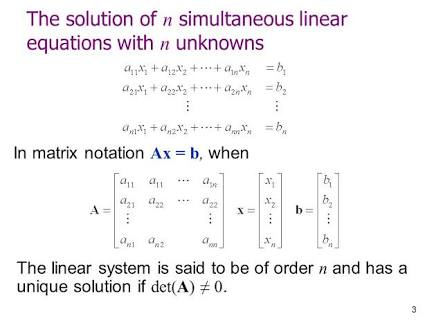# The number of values of   for which the system of linear equations  has non-trival solution, is: Option 1)threeOption 2)fourOption 3)twoOption 4)one

Homogeneous system of linear equation -

- whereinAs we have learnt from the concept for non-trivial solution

Option 1)

three

Option 2)

four

Option 3)

two

Option 4)

one

Exams
Articles
Questions